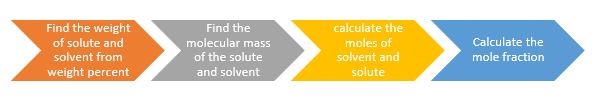# Weight Percent Formula | How to convert weight percent to mole fraction

In this post , we will be checking out what is weight percent or mass percent,what is Weight percent or mass percent formula, how to convert weight percent to mole fraction

Weight percent or mass percent is defined as the weight of the solute in 100 gm of the solution

$Weight \; percent= \frac {mass \; of \; the \; solute}{mass \; of \; the \; solution} \times 100$

So , if m is the mass of Solute and M is the mass of solvent, then Weight percent formula will be

$Weight \; percent= \frac {m}{m+M} \times 100$

It is generally given as 30% NaCL by weight solution or 50% HCL by weight solution

Example on Weight percent

1. A solution is prepared by adding 3 g of a substance A to 17 g of water. Calculate the mass per cent of the solute.

Solution

$Weight \; percent= \frac {mass \; of \; the \; solute}{mass \; of \; the \; solution} \times 100$

$=\frac {3}{3+17} \times 100$

=15%

2. How many grams of 5.0% by weight NaCL solutions are required to 6.4 g NaCL?

Solution

A 5% by weight NaCL solutions contains 5 g of NaCL in 100 g of solution

So 1 g of NaCL will be contained in = 100/5 =20 g of solution

Therefore 6.4 g of NaCL will be present in = 20 X 6.4 = 128 g of solution

Alternate Method

$Weight \; percent= \frac {mass \; of \; the \; solute}{mass \; of \; the \; solution} \times 100$

$5= \frac {6.4}{x} \times 100$

or x = 128 g

How to convert Weight percent to mole fraction

Now if weight percent of the solution is known, we can calculate the mole fraction of the solution or the solvent easily as explained with below example

Example

Calculate the mole fraction of HCl and H2O in a solution of HCL acid in water, containing 20% HCl by weight.
Solution
The solution contains 20 grams of HCL acid and 80 grams of water. Also,
Molar mass of HCl is 36.5 grams/mole.
Molar mass of water is 18 grams/mole.
Moles of HCl = 20/36.5 = .547 moles of HCl
Moles of water = 80/18 = 4.44 moles of H2O.

$Mole \; Fraction \; of \; solute = \frac {no \; of \; moles \; of \; solute}{no \; of \; moles \; of \; solute + no \; of \; moles \; of \; solvent}$
$Mole \; fraction \; of \; HCl = \frac {.547}{.547 + 4.44}= .109$
$Mole fraction of H2O = \frac {4.44}{.547 + 4.44} = .891$

So in short, following steps need to be executed1. Find the weight of Solute and solvent from weight percent or mass percent
2. Find the molecules mass of the solute and solvent
3. Calculate the moles of solute and solvent using weight and molecular mass
4. Apply the mole fraction formula to obtain the mole fraction

Practice Questions

1.  Find the mass percent if  10 g of X is dissolved in  80 g of Y?
2. Calculate the mole fraction of HCl and H2O in a solution of HCL acid in water, containing 10% HCl by weight.
3.  A solution is prepared by adding 2 g of substance to 18 g of water. Calculate the mass percent of solute
4. 20% (w/W) NaOH solution . Calculate the Mole fraction of NaOH and water

Related Articles

Molarity Formula

Molality Formula

### Search Our Website

Subscribe
Notify of

This site uses Akismet to reduce spam. Learn how your comment data is processed.

1 Comment
Inline Feedbacks